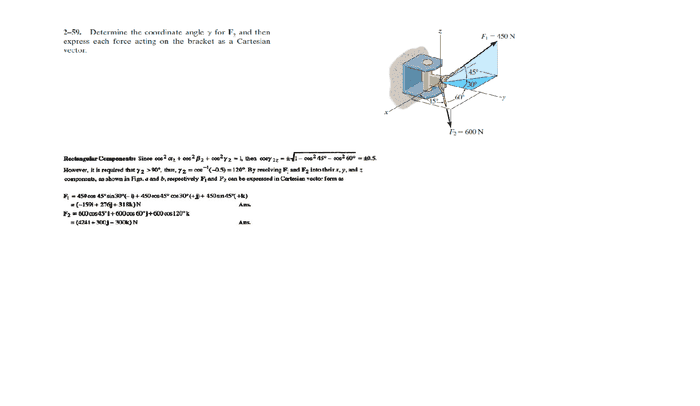# Engineering statics questions

• Mdhiggenz

## Homework Statement

Hello everyone,

I have a few questions regarding the problem below.

1. I did the process for solving γ correct however I took the 60° angle instead of the 120° angle. The below solution states that γ>90°. Why must is be greater, than 90°, and when would it have been smaller?

2. I got a big confused when solving for the projected portion of the triangle. What I did initially was solve for the hypotenuse of the projection which I thought would be

F'=450sin(45°), and just use that F' to find the x, and y components, however that was completely wrong it seems.## Homework Statement

Hello everyone,

I have a few questions regarding the problem below.

1. I did the process for solving γ correct however I took the 60° angle instead of the 120° angle. The below solution states that γ>90°. Why must is be greater, than 90°, and when would it have been smaller?
Where is γ measured from?
If F2 pointing above pr below the x-y plane?

2. I got a big confused when solving for the projected portion of the triangle. What I did initially was solve for the hypotenuse of the projection which I thought would be

F'=450sin(45°), and just use that F' to find the x, and y components, however that was completely wrong it seems.
F1 forms the hypotenuse of a 45deg triangle, Is this the one you mean?

How did you get the x and y components from there?

Note: the angles are very friendly.
i.e. sin(45)=cos(45)=1/√2, sin(30)=cos(30)=1/2, etc.

-------------------------

[*] except it's the cosine - doesn't matter to the result though.
Does matter for the next results.

Last edited:
γ is measured from the z axis to F2. It seems that F2 is below the xy plane. For the projection what I mean was for the 30degree the way my mind analyzes it is that we get the x components of both triangles e.g 30 and 45 degree.

Which would mean that 450*cos(45) would be the x component for the 45 degree right triangle, and cos(30) would be for the 30 degree triangle.

Which would make the i comonent. 450*cos(45)cos(30), however the solution above has it as 450*cos(45) sin(30)

γ is measured from the z axis to F2. It seems that F2 is below the xy plane.
The below solution states that γ>90°. Why must is be greater, than 90°, and when would it have been smaller?

If F2 were exactly in the x-y plane, what would γ equal?
Since it is below the x-y plane, would γ be bigger or smaller than this value?

For the projection what I mean was for the 30degree the way my mind analyzes it is that we get the x components of both triangles e.g 30 and 45 degree.

Which would mean that 450*cos(45) would be the x component for the 45 degree right triangle, and cos(30) would be for the 30 degree triangle.

Which would make the i comonent. 450*cos(45)cos(30), however the solution above has it as 450*cos(45) sin(30)

Looks like you got the sines and cosines mixed up.
You got it right for if the 30deg angle was measured from the +x axis like normal - but it isn't.
It can help if you sketch the triangle out separately and label the sides before working out which is the adjacent and which the opposite sides.

Oh because since it is below the xy plane that would mean it would be in either quadrants 3 or 4, but would that mean it should be γ>180 not 90?. I do understand though that the max a triangle can be is 180 I just don't understand that relation.

For the projection of the 30 degree triangle I now see that it is on the yz plane. But still don't see how that would help me. Even if I labeled the sides, for example I know that cos(30) would be on the y-axis now. But I can't see how that is in any way related to the X axis.

Oh because since it is below the xy plane that would mean it would be in either quadrants 3 or 4, but would that mean it should be γ>180 not 90?. I do understand though that the max a triangle can be is 180 I just don't understand that relation.
Careful: ##\gamma## cannot be bigger than 180deg.

What is the angle between the z-axis and anywhere on the x-y plane?

For the projection of the 30 degree triangle I now see that it is on the yz plane.
It isn't. But the angle is to the y-axis in the x-y plane. The formula ##x=r\cos\theta## only works for when ##\theta## is measured from the x axis.

But still don't see how that would help me. Even if I labeled the sides, for example I know that cos(30) would be on the y-axis now. But I can't see how that is in any way related to the X axis.
If ##\cos(30)## is on the y axis, then what is the trig function that would be on the x axis?